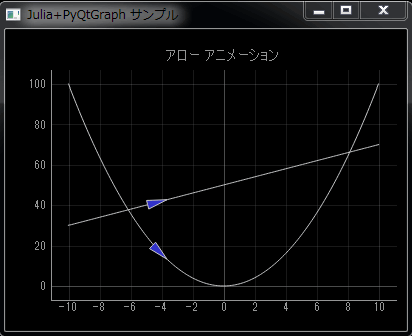# [PYTHON] Draw a graph with Julia + PyQtGraph (1)

"Draw a graph in the programming language Julia" I succeeded in moving the sample for the time being, but the data created by my own program (in Julia language) I decided to find out how to draw a graph using. I made a graph of a simple function and a sample of arrow animation. As usual, the calculation result obtained by Julia is displayed as a graph in PyQtGraph. (Please create an environment and move it)

• ArrowSample.jl (Julia source code) passes an array to a function called graph_run () in the Python source. Let Python draw the graph for you. Calculate \$ y \$ of \$ y = x ^ 2 \$ and \$ y = 2x + 50 \$ in the range of \$ -10 ≤ x ≤ 10 \$ (\$ x \$ in 0.1 increments).
• ArrowSample.py (Python source code) draws a line graph based on the array passed by Julia.
• When calling Python code using PyCall, C: \ julia-0.2.1-win32 \ bin is included in the load path, so both ArrowSample.jl and ArrowSample.py should be C: \ julia- Save it under 0.2.1-win32 \ bin.
• When saving the source code, the character encoding should be utf-8.
• To launch the sample, double-click ArrowSample.jl (Julia source code) from Explorer.

Note: For the environment construction, refer to "Drawing a graph in the programming language Julia".#### `jl:ArrowSample.jl(C:\julia-0.2.1-win32\Save to bin)`

``````
# -*- coding: utf-8 -*-

using PyCall

@pyimport ArrowSample
x  = [ -10 : 0.1 : 10 ]
y1 = [ x[i]*x[i] for i=1:length(x) ]
y2 = [ x[i]*2+50 for i=1:length(x) ]
ArrowSample.graph_run(x, y1, y2)
``````

#### `python:ArrowSample.py(C:\julia-0.2.1-win32\Save to bin)`

``````
# -*- coding: utf-8 -*-

from pyqtgraph.Qt import QtGui, QtCore
import pyqtgraph as pg

def graph_run(plot_data_x, plot_data_y1, plot_data_y2):

app = QtGui.QApplication([])

win = pg.GraphicsWindow(title=u"Julia+PyQtGraph sample")
win.resize(400,300)

# Enable antialiasing for prettier plots
pg.setConfigOptions(antialias=True)

c1 = p1.plot(x=plot_data_x, y=plot_data_y1)
c2 = p1.plot(x=plot_data_x, y=plot_data_y2)
p1.showGrid(x=True, y=True)

a1 = pg.CurveArrow(c1)